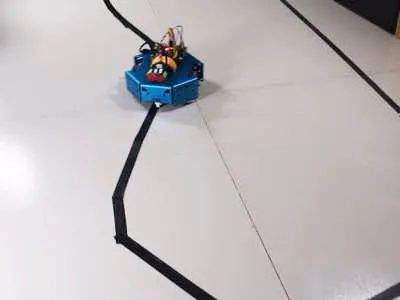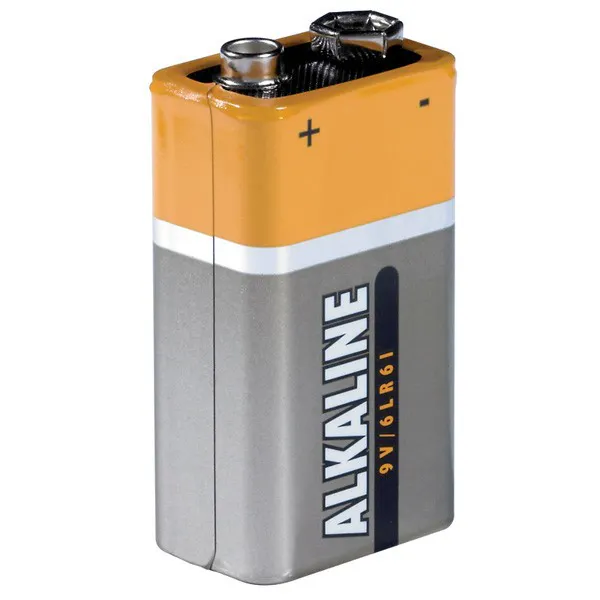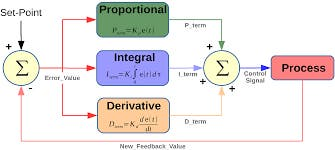Project tutorial# PID Control Line Follower Robot © MIT

Cool robot that follows black line. Learn and make a line follower robot with PID controller in 2 hours.

• 37,601 views
• 42 respects

## Components and supplies

 KittenBot Anodized Aluminum Chassis
×1
 KittenBot RosBot Baseboard
×1
 KittenBot Diameter Ball Caster with Metal Ball (Pair)
×1
 KittenBot Rubber Wheel with Motor Hub Set (Pair)
×1
 KittenBot 5-Channel IR Line-follwing Tracker Sensor
×1
 DC motor (generic)
×19V battery (generic)
×1Arduino UNO
×1

## Apps and online services

### Overview

One feature that most rover autonomous robots need is line following. The purpose of this project is to build a line follower robot and get started on learning PID controller in a fun way.

### Parts

The robot function properly with two motors, the Rosbot Baseboard, and a 5-Channel sensor. Unlike others, you don't have to buy extra H-bridge motor driver or various components since the Rosbot Baseboard has in-built 2x H-bridge dual driver. Simply connects motors to the Rosbot baseboard and it will supply more power than Arduino Uno.

Cool and solid chassis that has tons of mounting holes (4.8mm LEGO Technic), you can definitely reuse this chassis for other fun projects.

An Arduino UNO based mainboard with 2x on-board dual H-bridge motor drivers.

5-Channel Infrared detector, more accurate and stable.

### Step 1: Assembly

This robot is fairly easy to assemble, follow the instruction and it takes you about 15 minutes.

First, attach your motors to the sides of chassis, simply plug in the rubber wheel.

Mount the 5-Channel IR sensor to the front of the chassis.

Attach your Rosbot baseboard to the chassis, then the robot is ready to get wired up.

### Step 2: Wring

The following are the connections for 5-Channel IR sensor:

• VCC to 5V
• GND to RosBot's GND
• T1-T4 to pin A0-A3
• T5 to pin SDA

DC motors simply go to pin A+A- and pin B+B-.

### Coding

In the codes, we have a state machine that indicates each possible sensor array output. The robot moves to a certain direction according to the sensor array output.

``````void stateMachine(int a) {
switch (a) {
case B00000:
outlineCnt++;
break;
case B11111:
outlineCnt++;
break;
case B00010:
case B00110:
outlineCnt = 0;
pixels.setPixelColor(2, pixels.Color(0, 50, 0));
bias = 1;
break;
case B00001:
case B00011:
outlineCnt = 0;
pixels.setPixelColor(2, pixels.Color(0, 200, 0));
bias = 2;
break;
case B00100:
outlineCnt = 0;
pixels.setPixelColor(2, pixels.Color(0, 0, 20));
bias = 0;
break;
case B01000:
case B01100:
outlineCnt = 0;
pixels.setPixelColor(2, pixels.Color(50, 0, 0));
bias = -1;
break;
case B10000:
case B11000:
outlineCnt = 0;
pixels.setPixelColor(2, pixels.Color(200, 0, 0));
bias = -2;
break;
default:
Serial.println(a,BIN);
outlineCnt++;
break;
}
``````

We already set up the value of Error, proportion term, integral term, and derivative term.

``````float Kp = 25;
float Ki = 0.15;
float Kd = 1200;
float error, errorLast, erroInte;
float calcPid(float input) {
float errorDiff;
float output;
error = error * 0.7 + input * 0.3; // filter
//error = input;
errorDiff = error - errorLast;
erroInte = constrain(erroInte + error, -50, 50);
output = Kp * error + Ki * erroInte + Kd * errorDiff;
Serial.print(error); Serial.print(' ');
Serial.print(erroInte); Serial.print(' ');
Serial.print(errorDiff); Serial.print(' ');
Serial.println(output);
errorLast = error;
return output;
``````

Manipulate the values to find the best fit for your robot.

## Code

##### Line follower robotArduino
In the codes, we include a NeoPixel from Adafruit, but that's optional.
```#include <Adafruit_NeoPixel.h>
#define S_NULL 0
#define S_ONTRACE 1

void doDcSpeed(int spdL, int spdR) {
spdR = -spdR;
if (spdL < 0) {
analogWrite(5, 0);
analogWrite(6, -spdL);
} else {
analogWrite(5, spdL);
analogWrite(6, 0);
}

if (spdR < 0) {
analogWrite(9, 0);
analogWrite(10, -spdR);
} else {
analogWrite(9, spdR);
analogWrite(10, 0);
}
}

int bias = 0;
int outlineCnt = 0;

void stateMachine(int a) {
switch (a) {
case B00000:
outlineCnt++;
break;
case B11111:
outlineCnt++;
break;
case B00010:
case B00110:
outlineCnt = 0;
pixels.setPixelColor(2, pixels.Color(0, 50, 0));
bias = 1;
break;
case B00001:
case B00011:
outlineCnt = 0;
pixels.setPixelColor(2, pixels.Color(0, 200, 0));
bias = 2;
break;
case B00100:
outlineCnt = 0;
pixels.setPixelColor(2, pixels.Color(0, 0, 20));
bias = 0;
break;
case B01000:
case B01100:
outlineCnt = 0;
pixels.setPixelColor(2, pixels.Color(50, 0, 0));
bias = -1;
break;
case B10000:
case B11000:
outlineCnt = 0;
pixels.setPixelColor(2, pixels.Color(200, 0, 0));
bias = -2;
break;
default:
Serial.println(a,BIN);
outlineCnt++;
break;
}

pixels.setPixelColor(0, pixels.Color(outlineCnt * 10, 0, 0));
if (outlineCnt > 10) {
doDcSpeed(0,0);
} else {
float ff = 150;
float ctrl = calcPid(bias);
doDcSpeed(ff-ctrl,ff+ctrl);
}
pixels.show();
}

float Kp = 25;
float Ki = 0.15;
float Kd = 1200;
float error, errorLast, erroInte;

float calcPid(float input) {
float errorDiff;
float output;
error = error * 0.7 + input * 0.3; // filter
//error = input;
errorDiff = error - errorLast;
erroInte = constrain(erroInte + error, -50, 50);
output = Kp * error + Ki * erroInte + Kd * errorDiff;
Serial.print(error); Serial.print(' ');
Serial.print(erroInte); Serial.print(' ');
Serial.print(errorDiff); Serial.print(' ');
Serial.println(output);
errorLast = error;

return output;
}

int echoTrace() {
int ret = 0;
int a;
for (int i = 0; i < 5; i++) {
a[i] = constrain((1025 - analogRead(A0 + i)) / 10 - 4, 0, 20);
if (a[i] > 2) ret += (0x1 << i);
}
return ret;
}

void setup() {
Serial.begin(115200);
pixels.begin();
}

int pos;
void loop() {
delay(5);
pos = echoTrace();
stateMachine(pos);

}
```

## Schematics#### Line Follower Robot - PID Control - Android Setup

Project tutorial by MJRoBot

• 36,286 views
• 46 respects

#### Elliot the Line Follower Robot

Project showcase by SMM2

• 11,412 views
• 26 respects

#### Easiest Line Follower Robot

Project tutorial by Zubayer Al Billal Khan

• 35,634 views
• 53 respects

#### MeArm Robot Arm - Your Robot - V1.0

Project tutorial by Benjamin Gray

• 29,566 views
• 42 respects

#### How to Make a Gesture Control Robot at Home

Project tutorial by Shubham Shinganapure

• 10,261 views